# Travel Map With Cartopy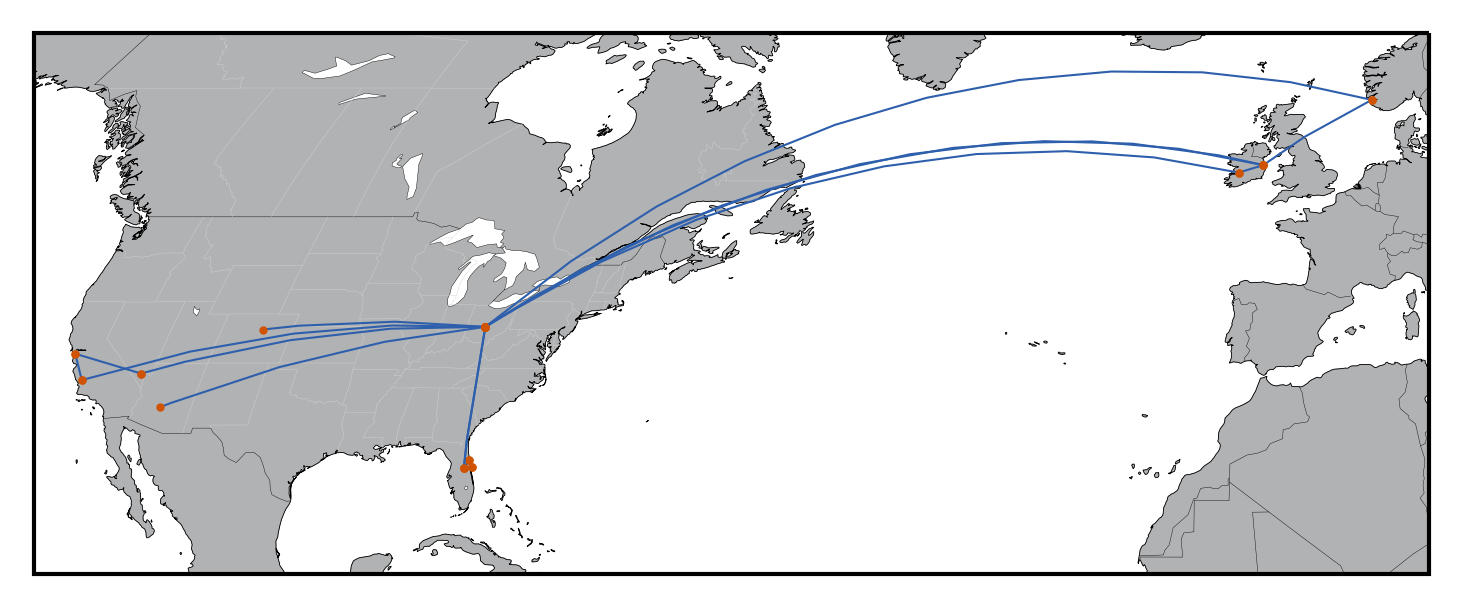In a former post I generated a travel map plotted with Basemap. From the Basemap documentation, however, Cartopy will replace Basemap:

Starting in 2016, Basemap came under new management. The Cartopy project will replace Basemap, but it hasn’t yet implemented all of Basemap’s features. All new software development should try to use Cartopy whenever possible, and existing software should start the process of switching over to use Cartopy. All maintenance and development efforts should be focused on Cartopy.

In this post, I generate a similar travel map using Cartopy.

In :
%matplotlib inline
import matplotlib
import cartopy
import pandas as pd

In :
%load_ext watermark
%watermark -iv

matplotlib  2.2.2
cartopy     0.16.0
pandas      0.22.0



We start with a simple example from the Cartopy documentation to plot a ground track between two points.

In :
import matplotlib.pyplot as plt
import cartopy.crs as ccrs

ax = plt.axes(projection=ccrs.Robinson())
ax.set_global()
ax.coastlines()

# [lng1, lng2], [lat1, lat2]
plt.plot([-6.26, -82.99], [53.35, 39.96], color='blue',
transform=ccrs.PlateCarree())

Out:
[<matplotlib.lines.Line2D at 0x1815568898>]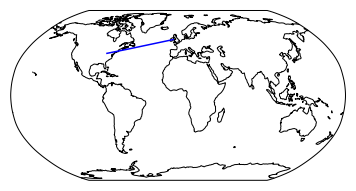Switching from the PlateCarree transformation to the Geodetic transformation, we can instead plot a great circle between to points.

In :
import matplotlib.pyplot as plt
import cartopy.crs as ccrs

ax = plt.axes(projection=ccrs.Robinson())
ax.set_global()
ax.coastlines()

# [lng1, lng2], [lat1, lat2]
plt.plot([-6.26, -82.99], [53.35, 39.96], color='#2E5FAC',
transform=ccrs.Geodetic())

Out:
[<matplotlib.lines.Line2D at 0x181561fcf8>]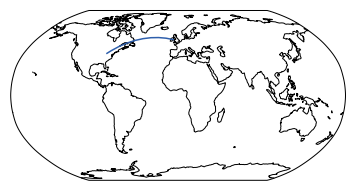Next we shade the land and water areas. Note that the water defaults to white so line 12 to specify white water is redundant, but I leave this in should you choose to use a different color. I will later remove this when increasing the resolution of the coastlines.

In :
import matplotlib.pyplot as plt
import cartopy.crs as ccrs
import cartopy

ax = plt.axes(projection=ccrs.Robinson())
ax.set_global()
ax.coastlines()

# color land and water
ax.add_feature(cartopy.feature.LAND, zorder=0, edgecolor='#7f7f7f',
facecolor='#B1B2B4')
ax.add_feature(cartopy.feature.OCEAN, zorder=0, facecolor='white')

# [lng1, lng2], [lat1, lat2]
plt.plot([-6.26, -82.99], [53.35, 39.96], color='#2E5FAC',
transform=ccrs.Geodetic())

Out:
[<matplotlib.lines.Line2D at 0x18156a8a58>]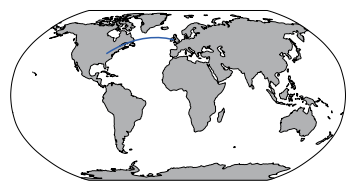Next we use set_extent to zoom in on the area of interest.

In :
%matplotlib inline
import matplotlib.pyplot as plt
import cartopy.crs as ccrs
import cartopy

ax = plt.axes(projection=ccrs.Robinson())

ax.set_global()
ax.coastlines()

# [lon_min, lon_max, lat_min, lat_max]
ax.set_extent([-120, 10, 20, 65], crs=ccrs.PlateCarree())

# color land and water
ax.add_feature(cartopy.feature.LAND, zorder=0, edgecolor='#7f7f7f',
facecolor='#B1B2B4')
ax.add_feature(cartopy.feature.OCEAN, zorder=0, facecolor='white')

# [lon1, lon2], [lat1, lat2]
plt.plot([-6.26, -82.99], [53.35, 39.96], color='#2E5FAC', marker='o',
transform=ccrs.Geodetic())

Out:
[<matplotlib.lines.Line2D at 0x18157ef748>]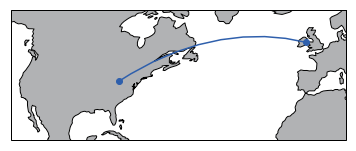Next we add country and state borders.

In :
%matplotlib inline
import matplotlib.pyplot as plt
import cartopy.crs as ccrs
import cartopy.feature as cfeature
import cartopy

ax = plt.axes(projection=ccrs.Robinson())

ax.set_global()
ax.coastlines()

# [lon_min, lon_max, lat_min, lat_max]
ax.set_extent([-120, 10, 20, 65], crs=ccrs.PlateCarree())

# color land and water
ax.add_feature(cartopy.feature.LAND, zorder=0,
edgecolor='#7f7f7f', facecolor='#B1B2B4')
ax.add_feature(cartopy.feature.OCEAN, zorder=0, facecolor='white')

# country borders
ax.add_feature(cartopy.feature.BORDERS, zorder=0,
edgecolor='#000000', linewidth=.1)

# state borders
states_provinces = cfeature.NaturalEarthFeature(
category='cultural',
name='admin_1_states_provinces_lines',
scale='50m',
facecolor='none', linewidth=.1)
ax.add_feature(states_provinces, edgecolor='#D3D3D3')

# [lon1, lon2], [lat1, lat2]
plt.plot([-6.26, -82.99], [53.35, 39.96], color='#2E5FAC',
marker='o', transform=ccrs.Geodetic())

Out:
[<matplotlib.lines.Line2D at 0x18157754a8>]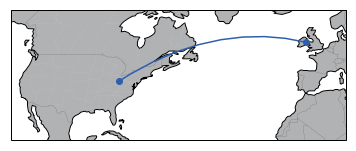Finally, we wrap this all up in a function, add lakes, increase the resolution of the land and coastlines, and load some data into a DataFrame to generate the plot.

In :
def plot_great_circles(df,fname):
import matplotlib.pyplot as plt
import cartopy.crs as ccrs
import cartopy.feature as cfeature
import cartopy

ax = plt.axes(projection=ccrs.Robinson())

ax.set_global()
ax.add_feature(cfeature.LAKES, facecolor='white',
edgecolor='black', linewidth=.1)
ax.coastlines('50m', linewidth=0.2)

# [lon_min, lon_max, lat_min, lat_max]
ax.set_extent([-120, 10, 20, 65], crs=ccrs.PlateCarree())

# add and color high-resolution land
LAND_highres = cfeature.NaturalEarthFeature('physical', 'land', '50m',
edgecolor='white',
facecolor='#B1B2B',
linewidth=.1
)
ax.add_feature(LAND_highres, zorder=0,
edgecolor='#7f7f7f', facecolor='#B1B2B4')

# add country borders
ax.add_feature(cartopy.feature.BORDERS, zorder=0,
edgecolor='#000000', linewidth=.1)

# add state borders
states_provinces = cfeature.NaturalEarthFeature(
category='cultural',
name='admin_1_states_provinces_lines',
scale='50m',
zorder=1,
facecolor='none',
linewidth=.1)
ax.add_feature(states_provinces, edgecolor='#D3D3D3')

# plot all great circles
for row in range(df.shape):
Lat_Orig = df.loc[df.index[row],'Lat_Orig']
Lng_Orig = df.loc[df.index[row],'Lng_Orig']
Lat_Des = df.loc[df.index[row],'Lat_Des']
Lng_Des = df.loc[df.index[row],'Lng_Des']
plt.plot([Lng_Orig, Lng_Des], [Lat_Orig, Lat_Des],
color='#2E5FAC', linewidth=.5,
marker='o', markerfacecolor='#CF5300',
markeredgecolor='#CF5300', markersize=1,
transform=ccrs.Geodetic())

plt.savefig(fname,bbox_inches='tight', dpi=300)

In :
df = pd.read_csv('2018_travel.csv')
df.head()

Out:
Name_Orig Lat_Orig Lng_Orig Name_Des Lat_Des Lng_Des
0 Columbus, OH 39.961 -82.999 Orlando, FL 28.538 -81.379
1 Orlando, FL 28.538 -81.379 Port Orange, FL 29.138 -80.995
2 Port Orange, FL 29.138 -80.995 KSC 28.573 -80.649
3 KSC 28.573 -80.649 Orlando, FL 28.538 -81.379
4 Columbus, OH 39.961 -82.999 Phoenix, AZ 33.448 -112.074

And here is our final plot:

In :
plot_great_circles(df,fname='2018_Travel.png')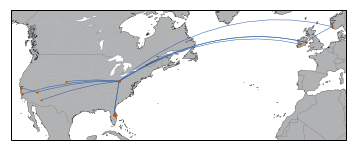## Closing Thoughts¶

Cartopy continues to be actively developed and I plan to use it for future cartographic plotting in Python. Also take a look at this great example of using Cartopy.

This post was written entirely in the Jupyter notebook. You can download the original notebook.

© 2005 Matthew Kudija | Source